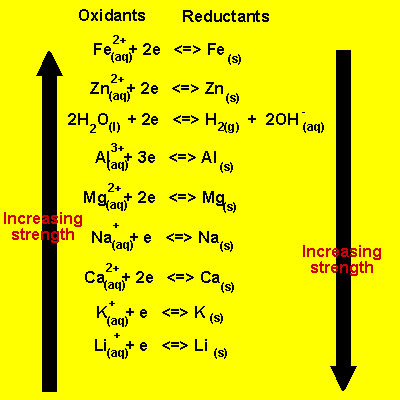## Predicting Spontaneous Direction of a Redox Reaction

#### Learning Objective

• Predict the direction of electron flow in a redox reaction given the reduction potentials of the two half-reactions

#### Key Points

• Sometimes, the direction of a redox reaction can be determined by estimating the relative strengths of the reductants and oxidants.
• In situations where an electrochemical series is not sufficient to absolutely determine the direction of a redox reaction, the standard electrode potential, Eo, can be used.
• A negative value of cell potential indicates a reducing environment, while a positive value indicates an oxidizing environment.

#### Term

• standard electrode potentialAn electrode potential measured under standard conditions (298 K, 1 atm, and 1 M).

## Predicting the Redox Half-Reactions

Generally, the direction of a redox reaction depends on the relative strengths of oxidants and reductants in a solution. In simple situations, an electrochemical series can be very useful for determining the direction of the reaction.Electrochemical seriesIn order to predict if two reactants will take part in a spontaneous redox reaction, it is important to know how they rank in an electrochemical series.

In the table provided, the most easily reduced element is Li and the most easily oxidized is iron. This means that Li would be written as the reduction half-reaction when compared to any other element in this table. On the other hand, Fe would be written as the oxidation half-reaction when compared to any other element on this table.

Some reactions cannot be “eyeballed” in this manner. These reactions require a more mathematical method to determine the direction. To figure this out, it is important to consider the standard electrode potential, which is a measure of the driving force behind a reaction. The sign of the standard electrode potential indicates the direction in which the reaction must shift to reach equilibrium.

Consider the reaction between zinc and acid:

$Zn(s) + 2 H^+(aq) \leftrightarrow Zn^{2+}(aq) + H_2(g)$

Oxidation: $Zn(s) \rightarrow Zn^{2+}(aq) + 2e^- \ E^o = 0.76\ V$

Reduction: $2H^+ + 2e^- \rightarrow H_2 \ E^o = 0.00\ V$

Eo = 0.76 V

The positive Eo value indicates that when this system is present at standard-state conditions, it has to shift to the right to reach equilibrium. Reactions for which Eo is positive, therefore, have equilibrium constants that favor the products of the reaction.

What happens to the standard electrode potential when the reaction is written in the reverse direction? Turning the reaction around doesn’t change the relative strengths of the oxidizing or reducing agents. The magnitude of the potential must remain the same. However, turning the equation around changes the sign of the standard electrode potential, and can therefore turn an unfavorable reaction into one that is spontaneous, or vice versa. For example if we turn the zinc oxidation half-reaction around ($Zn^{2+} + 2e^- \rightarrow Zn \ E^o = -0.76 V$), the cell potential is reversed.

The relative reactivities of different half-reactions can be compared to predict the direction of electron flow. Half-reaction equations can be combined if one is reversed to oxidation in a manner that cancels out the electrons.